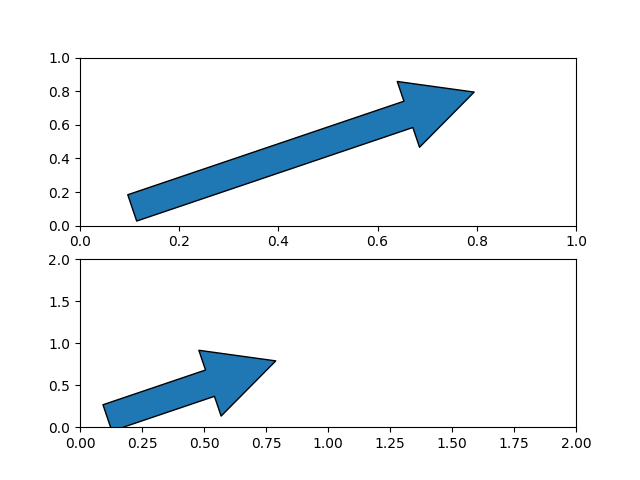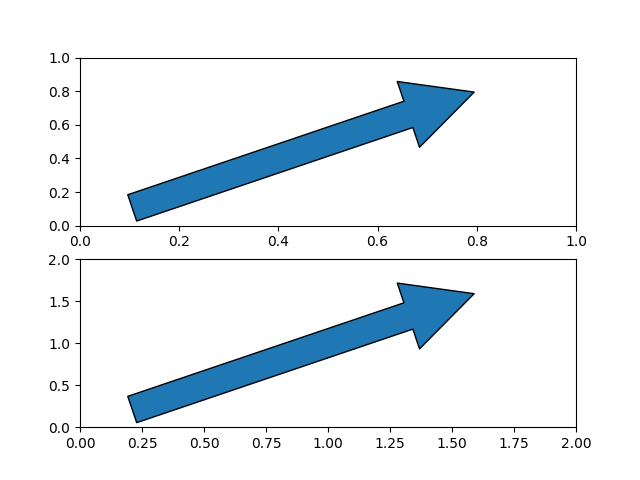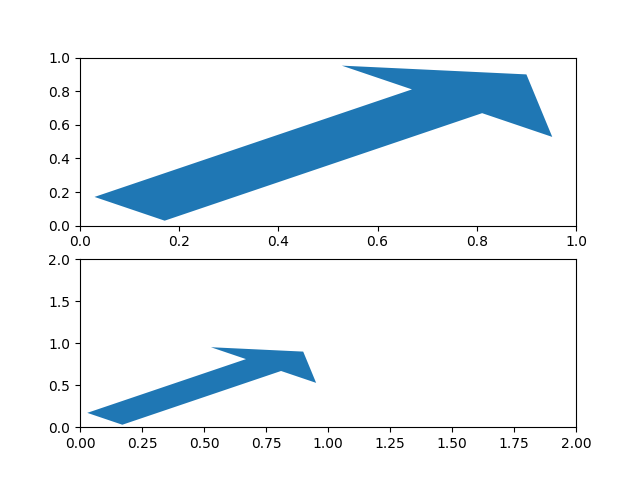# Arrow guide¶

Arrows are often used to annotate plots. This tutorial shows how to plot arrows that behave differently when the data limits on a plot are changed. In general, points on a plot can either be fixed in "data space" or "display space". Something plotted in data space moves when the data limits are altered - an example would the points in a scatter plot. Something plotted in display space stays static when data limits are altered - an example would be a figure title or the axis labels.

Arrows consist of a head (and possibly a tail) and a stem drawn between a start point and end point, called 'anchor points' from now on. Here we show three use cases for plotting arrows, depending on whether the head or anchor points need to be fixed in data or display space:

1. Head shape fixed in display space, anchor points fixed in data space
2. Head shape and anchor points fixed in display space
3. Entire patch fixed in data space

Below each use case is presented in turn.

import matplotlib.patches as mpatches
import matplotlib.pyplot as plt
x_tail = 0.1
y_tail = 0.1


## Head shape fixed in display space and anchor points fixed in data space¶

This is useful if you are annotating a plot, and don't want the arrow to to change shape or position if you pan or scale the plot. Note that when the axis limits change

In this case we use patches.FancyArrowPatch

Note that when the axis limits are changed, the arrow shape stays the same, but the anchor points move.

fig, axs = plt.subplots(nrows=2)
arrow = mpatches.FancyArrowPatch((x_tail, y_tail), (dx, dy),
mutation_scale=100)

arrow = mpatches.FancyArrowPatch((x_tail, y_tail), (dx, dy),
mutation_scale=100)
axs.set_xlim(0, 2)
axs.set_ylim(0, 2)Out:

(0.0, 2.0)


## Head shape and anchor points fixed in display space¶

This is useful if you are annotating a plot, and don't want the arrow to to change shape or position if you pan or scale the plot.

In this case we use patches.FancyArrowPatch, and pass the keyword argument transform=ax.transAxes where ax is the axes we are adding the patch to.

Note that when the axis limits are changed, the arrow shape and location stays the same.

fig, axs = plt.subplots(nrows=2)
arrow = mpatches.FancyArrowPatch((x_tail, y_tail), (dx, dy),
mutation_scale=100,
transform=axs.transAxes)

arrow = mpatches.FancyArrowPatch((x_tail, y_tail), (dx, dy),
mutation_scale=100,
transform=axs.transAxes)
axs.set_xlim(0, 2)
axs.set_ylim(0, 2)Out:

(0.0, 2.0)


## Head shape and anchor points fixed in data space¶

In this case we use patches.Arrow

Note that when the axis limits are changed, the arrow shape and location changes.

fig, axs = plt.subplots(nrows=2)

arrow = mpatches.Arrow(x_tail, y_tail, dx, dy)

arrow = mpatches.Arrow(x_tail, y_tail, dx, dy)
axs.set_xlim(0, 2)
axs.set_ylim(0, 2)Out:

(0.0, 2.0)

plt.show()


Total running time of the script: ( 0 minutes 1.033 seconds)

Keywords: matplotlib code example, codex, python plot, pyplot Gallery generated by Sphinx-Gallery